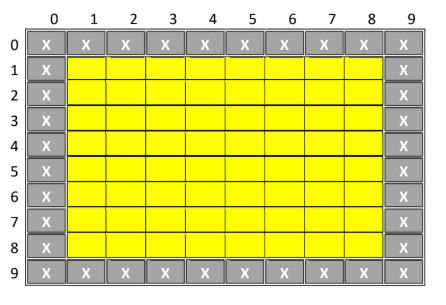top of page
Search

# C++ Assignment Help | Maze-Solving Program Assignment Help

Updated: Jun 13, 2021

Start with the computer maze-solving program covered in class. The source code is

posted along with the homework.

• Change the dimensions of the maze to 20x20 with walls around the borders. Out of the empty space in the middle, randomly fill 25% of them with walls. Then pick a random start location (that is empty) and a random Exit location (that is empty). Since you are using random numbers, you should get a different maze with a different start and end. There is a small chance that it is impossible to get from the start to the exit, but don't worry about that possibility (when the program runs it should just not print any solution).

• The algorithm we covered in class prints out the path from the start to the exit in reverse order. Modify the program so the path is output in forward order. One way to do this is to make an array (or arrays) that store the (x,y) coordinates of the current position as the recursive function exits. With all of the coordinates in an array, inside the main function you can now loop through the array in the proper order to output the moves from start to exit.

• Your program should output a solution to the randomly generated maze, if oneexists.

### Recursive Maze Generation

Implement the following recursive algorithm to randomly generate a maze. In the example below I have made the maze 10x10 to save space but your maze should be 40x40. Start with a 2D array of char like we used previously and assign walls to the borders:Call the recursive function with parameters that specify the blank area in the middle, in this case, highlighted by yellow below. You could do this a couple of ways, for example, pass in the coordinates of the upper left and lower right corner, or pass in the coordinate of the upper left corner plus the width and height of the area.If the width of the yellow area is <=2 cells or the height of the yellow area is <=2 cells then the function should return, doing nothing. This is the base case that terminates recursion.

If the height of the yellow area is greater than or equal to the width of the yellow area, then the yellow area is either square or taller than it is wide. In this case, the idea is to draw a horizontal wall somewhere in the green area:By avoiding the top and bottom row of the yellow area, we prevent ourselves from drawing a wall that might box ourselves in by covering up a passageway.

In the green area, randomly select a vertical position (in this case from y=2 to y=7) and draw a horizontal wall all the way across the green. In this example, I randomly selected y=3:Next, check if the ends of the line we just drew are next to a blank. If so, make the end wall a blank. This is to allow passage through the blank instead of blocking it. In this example, check if (0,3) is a blank. If it is, then make (1,3) a blank. Also check if (9,3) is a blank. If it is then make (8,3) a blank. There is no blank to add in this case.

Next, randomly select one of the cells in the wall that was added and turn it into a blank. This adds a hole to allow passage between the two halves of the maze separated by the new wall. In this example, I randomly picked x=3 and turned cell (3,3) into a blank:bottom of page